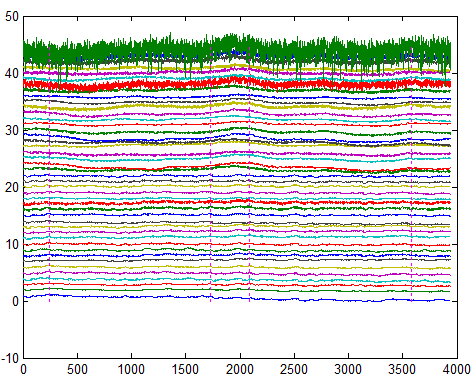# NIRS hyperscanning data analysis (3) quality check

Data quality check

1. Behavior data

To identify behavior abnormalities, we need to plot the behavior data for each individual subject. In this case, we plotted the reaction time vs trial. An example is shown below. It apparent that subject 2 (red) did something strange in trial 20.

We can also plot the difference of reaction time and the threshold to win. Obviously they only win 1 trial and this is unusual.

We can also find the mean, min and max of the reaction times. Below is the script:

```figure;plot([1:40],subjectData.reaction1,'s-')
hold on;plot([1:40],subjectData.reaction2,'s-r')

figure('color','w');plot([1:40],abs(subjectData.reaction1-subjectData.reaction2),'s-k')
hold on;plot([1:40],subjectData.winthreshold,'s-m')
legend({'reaction time difference','threshold'})
xlabel('trial');ylabel('second')

winNum = sum((subjectData.winthreshold - abs(subjectData.reaction1-subjectData.reaction2))>0);
disp('winning trials = ')
disp(winNum)

disp(length(subjectData.eventwarning))

disp(mean(subjectData.reaction1))
disp(max(subjectData.reaction1))
disp(min(subjectData.reaction1))

disp(mean(subjectData.reaction2))
disp(max(subjectData.reaction2))
disp(min(subjectData.reaction2))```

2. NIRS data

The 1st way to identify abnormalities in NIRS data is to plot all channel’s time series in one figure, like the figure below. In the following figure, the time series for each channel is plotted and aligned vertically. It’s easy to identify that the green channel (channel #44) has much more noise than others.Another way is to use wavelet transform. If the NIRS signal was acquired well, then the heart beating signal should be captured, leaving a bright brand in the frequency ~1Hz in the wavelet transform plot, just like the left plot in the figure below (the band close to period 8). If there is no such band, it does not necessarily mean the signal is trash, but you need to be cautious.

The third way is to check the correlation between hbo and hbr. They are supposed to have negative correlation. If not, or if they have perfect negative correlation (-1), then they might contain too much noise. We have a separate article on this method. Please check out https://www.alivelearn.net/?p=1767

```[hbo,hbr,mark]=readHitachData('SA06_MES_Probe1.csv');

figure;plotTraces(hbr,1:52,mark)

figure;wt(hbo(:,1))

for ii=1:52; wt(hbo(:,ii)); pause; end

3. Digitizer data

You want to make sure the measure digitizer data is reasonable by looking at the probe positions in a 3D space.

```pos_data=readPosFile('0001.pos');
figure;plot3(pos_data(:,1),pos_data(:,2),pos_data(:,3),'o');axis equal;```

 Don't want to miss new papers in your field? Check out Stork we developed:专注医学生物类的论文润色联系他们请用优惠码 STORK4，他们会给你折扣。## 第十九期 fNIRS Journal Club 通知 2021/05/29，9:30am